# DETAIL EVERY ANSWER WITH THE EXCEL FORMULA TO SOLVE THE EQUATION - EVERY ANSWER NEEDS AN EXCEL EQUATION DETAIL EVERY ANS...

DETAIL EVERY ANSWER WITH THE EXCEL FORMULA TO SOLVE THE EQUATION - EVERY ANSWER NEEDS AN EXCEL EQUATION

DETAIL EVERY ANSWER WITH THE EXCEL FORMULA TO SOLVE THE EQUATION - EVERY ANSWER NEEDS AN EXCEL EQUATION

DETAIL EVERY ANSWER WITH THE EXCEL FORMULA TO SOLVE THE EQUATION - EVERY ANSWER NEEDS AN EXCEL EQUATION

DETAIL EVERY ANSWER WITH THE EXCEL FORMULA TO SOLVE THE EQUATION - EVERY ANSWER NEEDS AN EXCEL EQUATION

DETAIL EVERY ANSWER WITH THE EXCEL FORMULA TO SOLVE THE EQUATION - EVERY ANSWER NEEDS AN EXCEL EQUATION

DETAIL EVERY ANSWER WITH THE EXCEL FORMULA TO SOLVE THE EQUATION - EVERY ANSWER NEEDS AN EXCEL EQUATION

DETAIL EVERY ANSWER WITH THE EXCEL FORMULA TO SOLVE THE EQUATION - EVERY ANSWER NEEDS AN EXCEL EQUATION

DETAIL EVERY ANSWER WITH THE EXCEL FORMULA TO SOLVE THE EQUATION - EVERY ANSWER NEEDS AN EXCEL EQUATION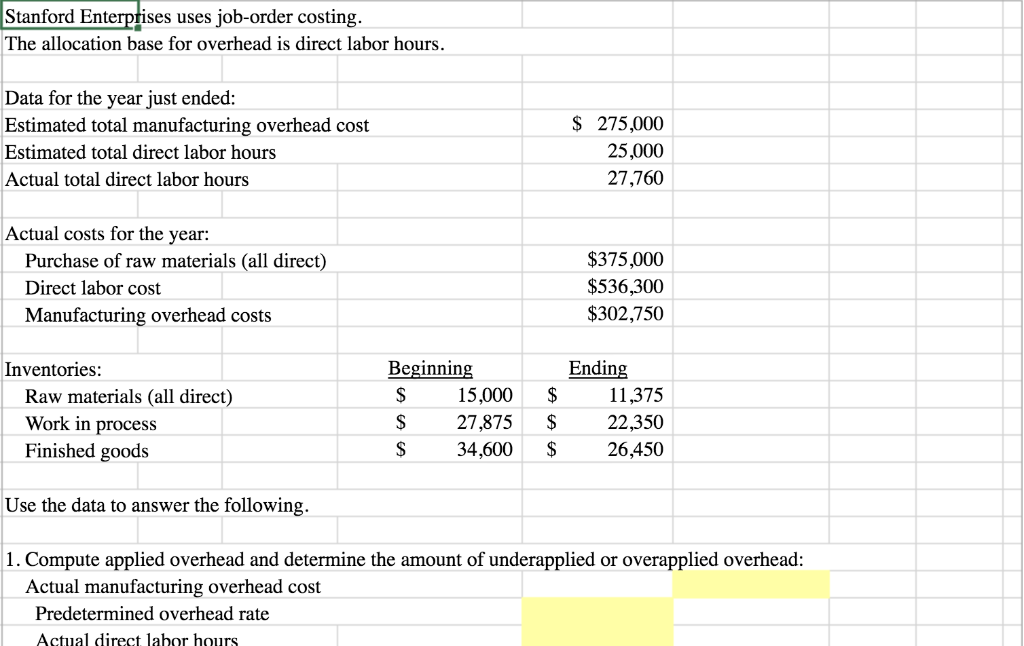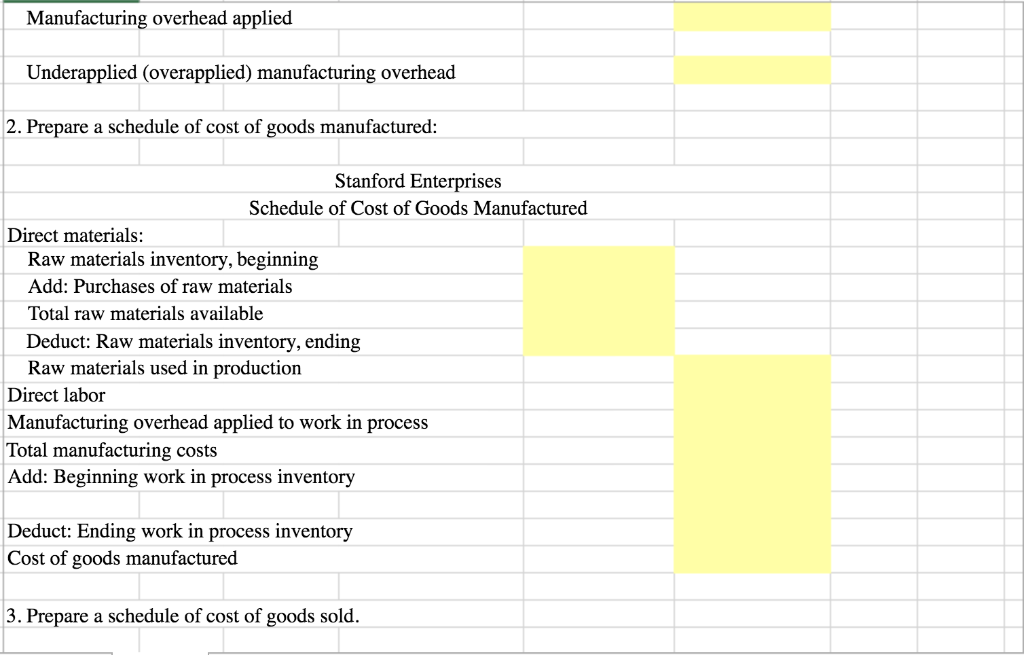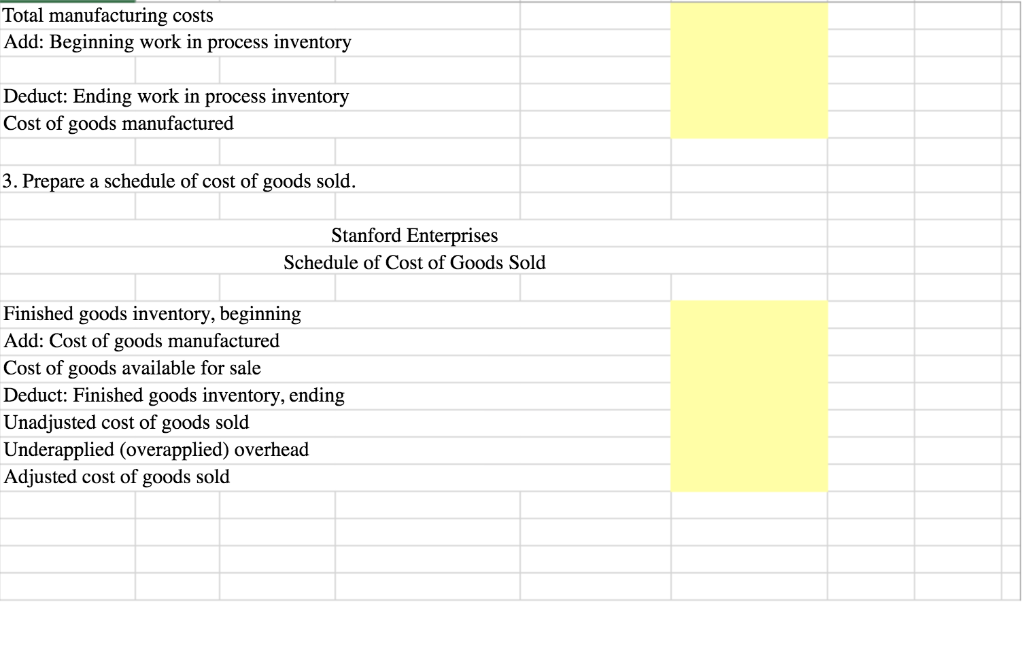Stanford Enterprises has provided its manufacturing estimated and actual data for the year end. The Controller has asked you to compute the predetermined overhead rate, the schedule of cost of goods manufactured, and the schedule of cost of goods sold. Use the information included in the Excel Simulation and the Excel functions described below to complete the task.

• Cell Reference: Allows you to refer to data from another cell in the worksheet. From the Excel Simulation below, if in a blank cell, “=E5” was entered, the formula would output the result from cell E5, or \$275,000 in this example.
• Basic Math functions: Allows you to use the basic math symbols to perform mathematical functions. You can use the following keys: + (plus sign to add), - (minus sign to subtract), * (asterisk sign to multiply), and / (forward slash to divide). From the Excel Simulation below, if in a blank cell “=E6+E7” was entered, the formula would add the values from those cells and output the result, or 52,760 in this example. If using the other math symbols the result would output an appropriate answer for its function.
• SUM function:  Allows you to refer to multiple cells and adds all the values. You can add individual cell references or ranges to utilize this function. From the Excel Simulation below, if in a blank cell “=SUM(E10,E11,E12)” was entered, the formula would output the result of adding those three separate cells, or 1,214,050 in this example. Similarly, if in a blank cell “=SUM(E10:E12)” was entered, the formula would output the same result of adding those cells, except they are expressed as a range in the formula, and the result would be 1,214,050 in this example.
Stanford Enterptises The allocation base for overhead is direct labor hours uses job-order costing Data for the year just ended: 275,000 Estimated total manufacturing overhead cost 25,000 Estimated total direct labor hours 27,760 Actual total direct labor hours Actual costs for the year: \$375,000 Purchase of raw materials (all direct) \$536,300 Direct labor cost \$302,750 Manufacturing overhead costs Beginning Ending Inventories: \$ 15,000 \$ 11,375 Raw materials (all direct) Work in process S \$ 22,350 27,875 Finished goods S \$ 34,600 26,450 Use the data to answer the following. 1. Compute applied overhead and determine the amount of underapplied or overapplied overhead: Actual manufacturing overhead cost Predetermined overhead rate Actual direct labor hours
Manufacturing overhead applied Underapplied (overapplied) manufacturing overhead 2. Prepare a schedule of cost of goods manufactured: Stanford Enterprises Schedule of Cost of Goods Manufactured Direct materials: Raw materials inventory, beginning Add: Purchases of raw materials Total raw materials available Deduct: Raw materials inventory, ending Raw materials used in production Direct labor Manufacturing overhead applied to work in process Total manufacturing costs Add: Beginning work in process inventory Deduct: Ending work in process inventory Cost of goods manufactured |3. Prepare a schedule of cost of goods sold
Total manufacturing costs Add: Beginning work in process inventory Deduct: Ending work in process inventory Cost of goods manufactured 3. Prepare a schedule of cost of goods sold. Stanford Enterprises Schedule of Cost of Goods Sold Finished goods inventory, beginning Add: Cost of goods manufactured Cost of goods available for sale Deduct: Finished goods inventory, ending Unadjusted cost of goods sold Underapplied (overapplied) overhead Adjusted cost of goods sold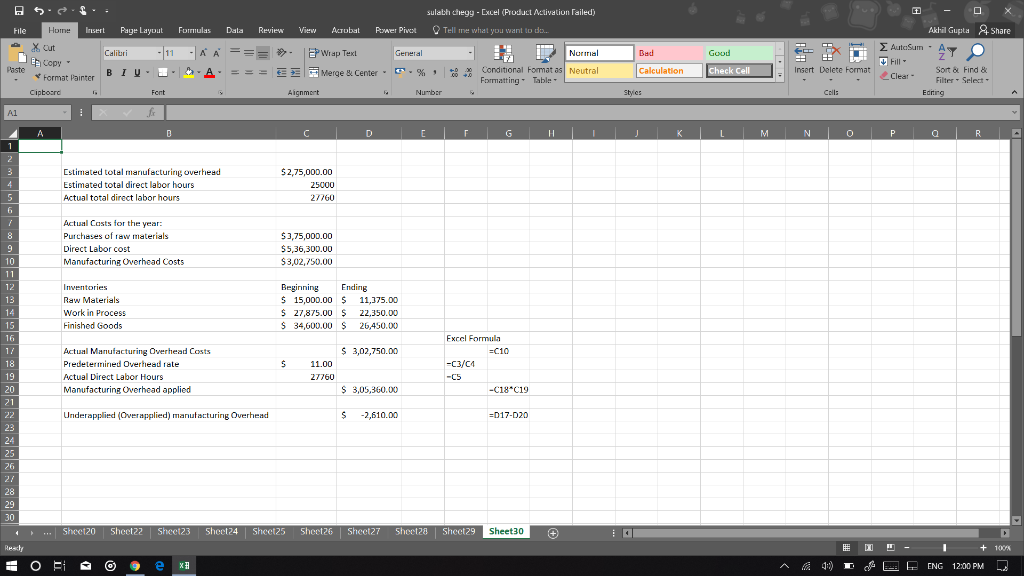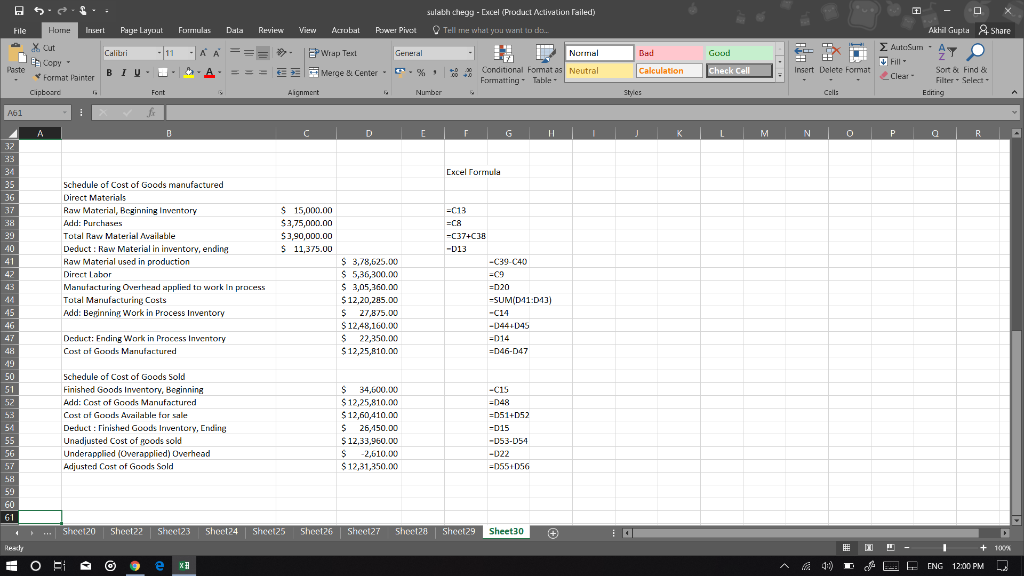##### Add Answer of: DETAIL EVERY ANSWER WITH THE EXCEL FORMULA TO SOLVE THE EQUATION - EVERY ANSWER NEEDS AN EXCEL EQUATION DETAIL EVERY ANS...
Similar Homework Help Questions
• ### Please give answers in the yellow boxes as an excel function and using cell references when...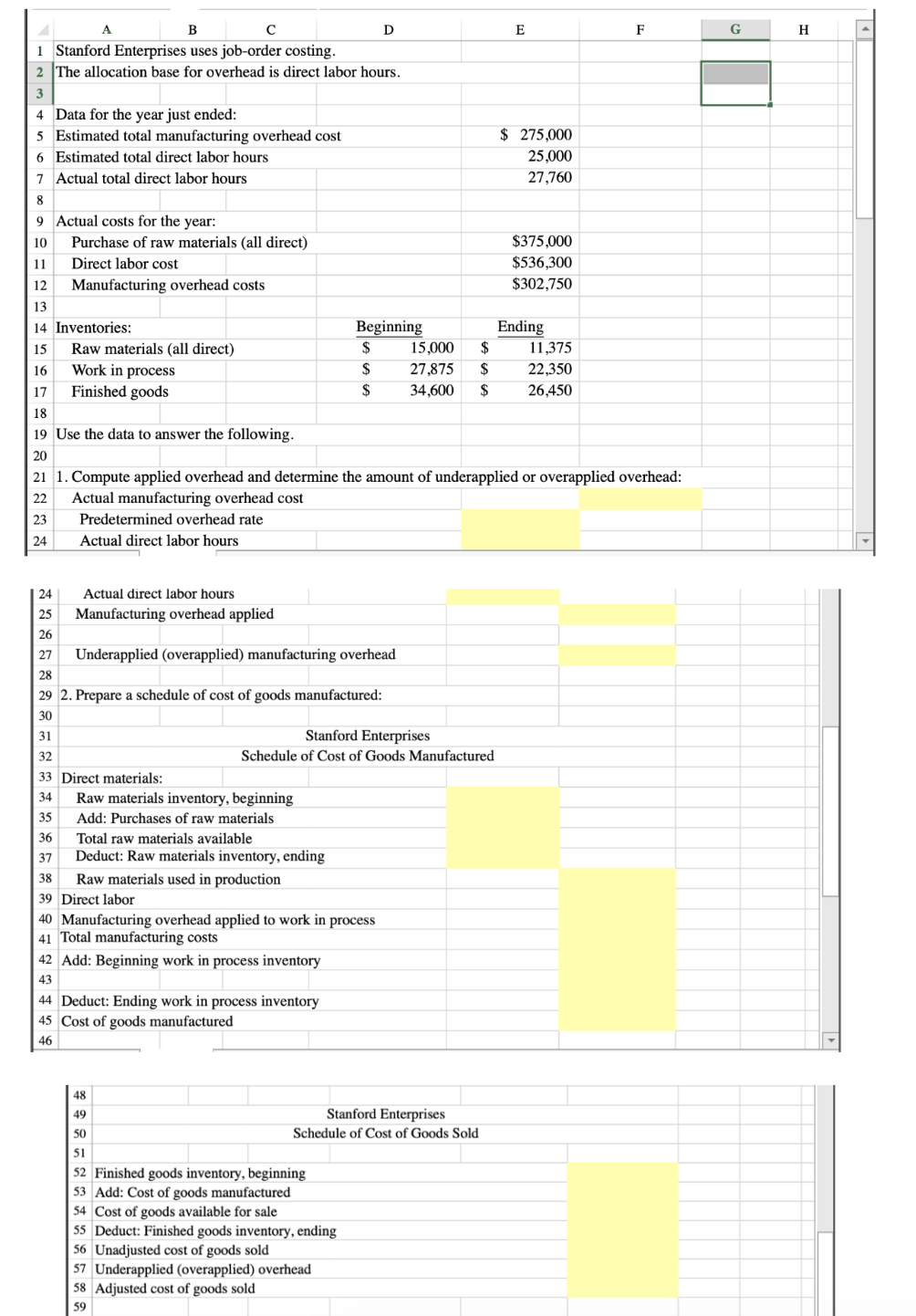Please give answers in the yellow boxes as an excel function and using cell references when needed. B F G H D i Stanford Enterprises uses job-order costing. 2 The allocation base for overhead is direct labor hours. 4 Data for the year just ended: 5 Estimated total manufacturing overhead cost 6 Estimated total direct labor hours 7 Actual total direct labor hours \$ 275,000 25,000 27,760 9 Actual costs for the year: 10 Purchase of raw materials (all direct)...

• ### Please give answers in the yellow boxes as an excel function and using cell references when...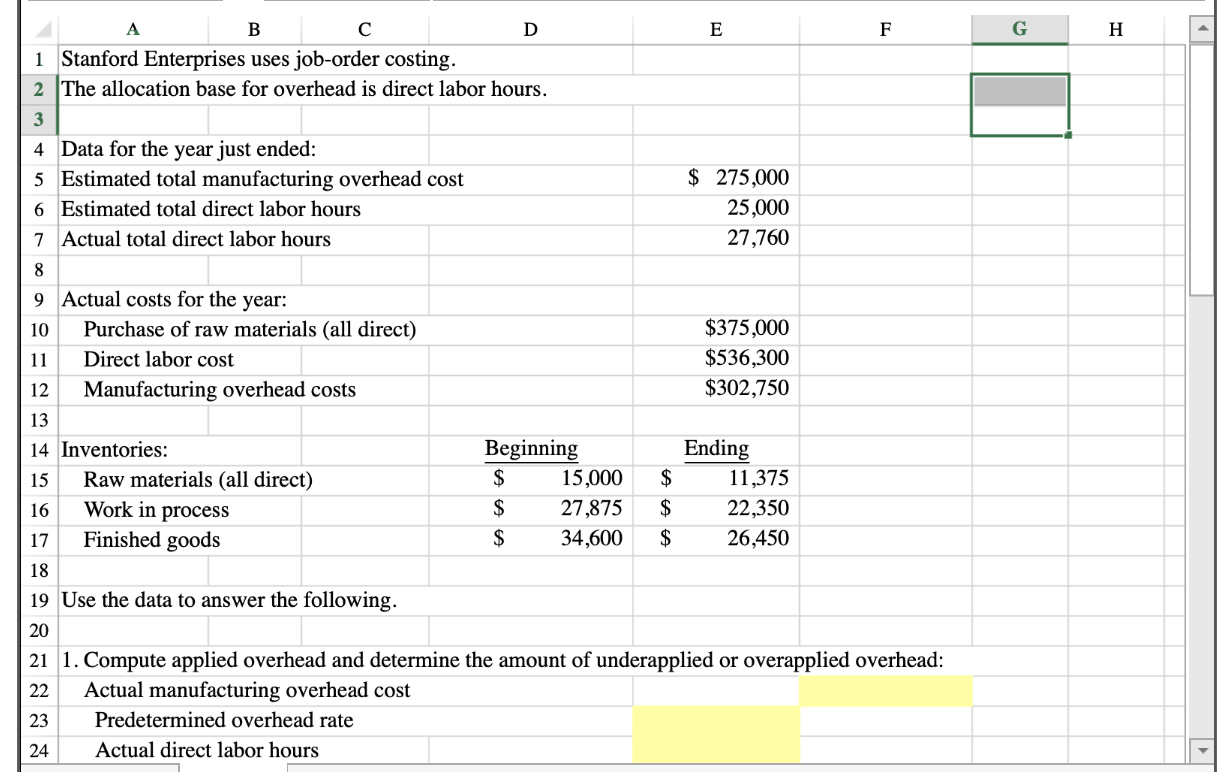Please give answers in the yellow boxes as an excel function and using cell references when needed. Н 1 Stanford Enterprises uses job-order costing. 2 The allocation base for overhead is direct labor hours. 3 4 Data for the year just ended: 5 Estimated total manufacturing overhead cost 6 Estimated total direct labor hours 7 Actual total direct labor hours \$ 275,000 25,000 27,760 9 Actual costs for the year: \$375,000 Purchase of raw materials (all direct) 10 \$536,300 \$302,750...

• ### Next to each answer, may you please include the equation that was used to find the...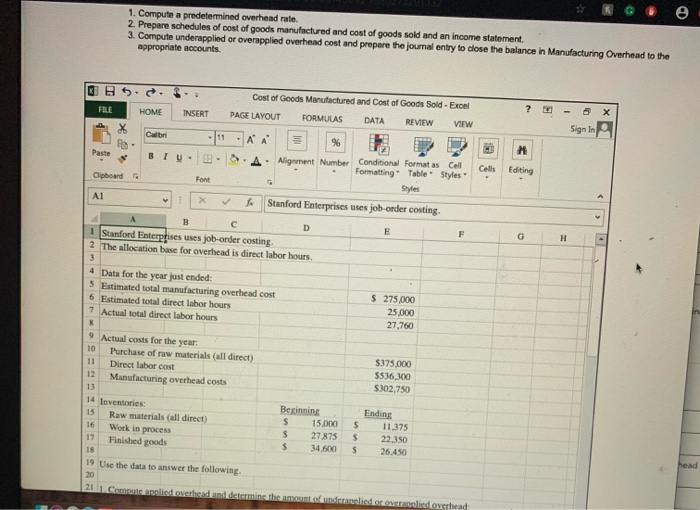Next to each answer, may you please include the equation that was used to find the solution. 1. Compute a predetermined overhead rate. 2. Prepare schedules of cost of goods manufactured and cost of goods sold and an income statement. 3. Compute underapplied or overapplied overhead cost and prepare the journal entry to dlose the balance in Manufacturing Overhead to the appropriate accounts. Cost of Goods Manufactured and Cost of Goods Sold - Excel FILE REVIEW HOME INSERT PAGE LAYOUT...

• ### Stanford Enterprises has provided its manufacturing estimated and actual data for the year end. The Controller...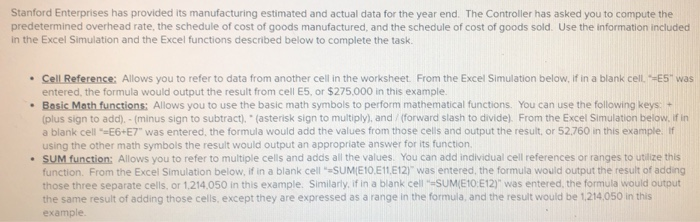Stanford Enterprises has provided its manufacturing estimated and actual data for the year end. The Controller has asked you to compute the predetermined overhead rate, the schedule of cost of goods manufactured, and the schedule of cost of goods sold. Use the information included in the Excel Simulation and the Excel functions described below to complete the task. • Cell Reference: Allows you to refer to data from another cell in the worksheet. From the Excel Simulation below. If in...

• ### please WRITE THE FORMULA NEXT TO the answers, dont answer my question without the formula (...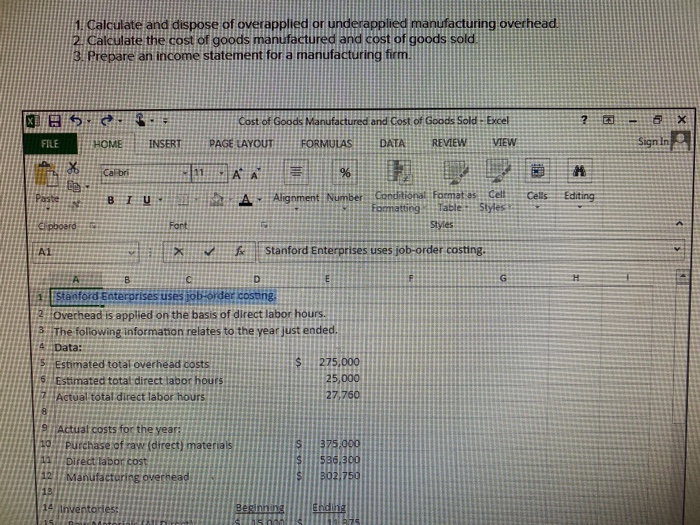please WRITE THE FORMULA NEXT TO the answers, dont answer my question without the formula ( I did some of this so just do the ones i didnt do in yellow) PLEASE please write the formulas. thanks 1. Calculate and dispose of overapplied or underapplied manufacturing overhead. 2. calculate the cost of goods manufactured and cost of goods sold, 3. Prepare an income statement for a manufacturing firm, Cost of Goods Manufactured and Cost of Goods Sold - Excel TAYOUT...

• ### Stanford Enterprises has provided its manufacturing estimated and actual data for the year end. The Controller...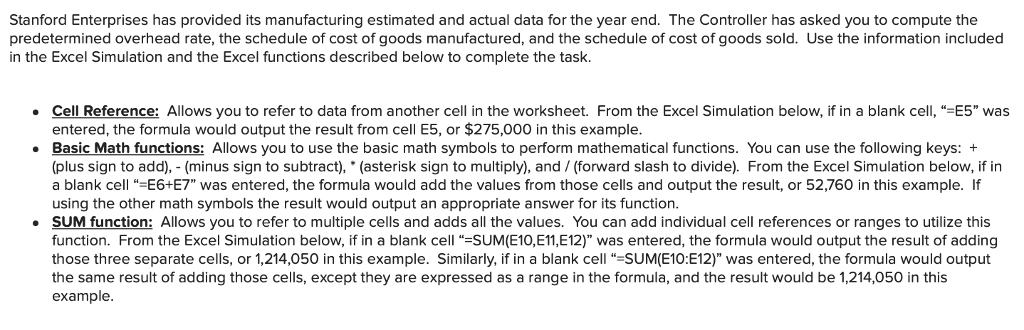Stanford Enterprises has provided its manufacturing estimated and actual data for the year end. The Controller has asked you to compute the predetermined overhead rate, the schedule of cost of goods manufactured, and the schedule of cost of goods sold. Use the information included in the Excel Simulation and the Excel functions described below to complete the task Cell Reference: Allows you to refer to data from another cell in the worksheet. From the Excel Simulation below, if in a...

• ### Compute a predetermined overhead rate. Prepare schedules of cost of goods manufactured and cost of goods...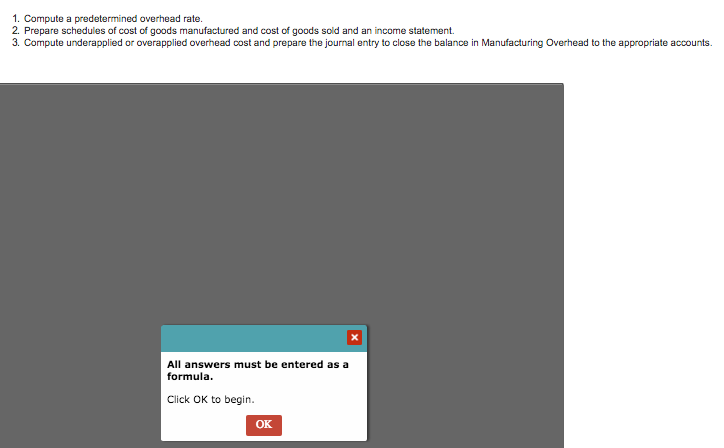Compute a predetermined overhead rate. Prepare schedules of cost of goods manufactured and cost of goods sold and an income statement. Compute underapplied or overapplied overhead cost and prepare the journal entry to close the balance in Manufacturing Overhead to the appropriate accounts. Says 'all answers must be entered as a formula' 1. Compute a predetermined overhead rate. 2. Prepare schedules of cost of goods manufactured and cost of goods sold and an income statement. 3. Compute underapplied or overapplied...

• ### Stanford Enterprises has provided its manufacturing estimated and actual data for the year end. The Controller...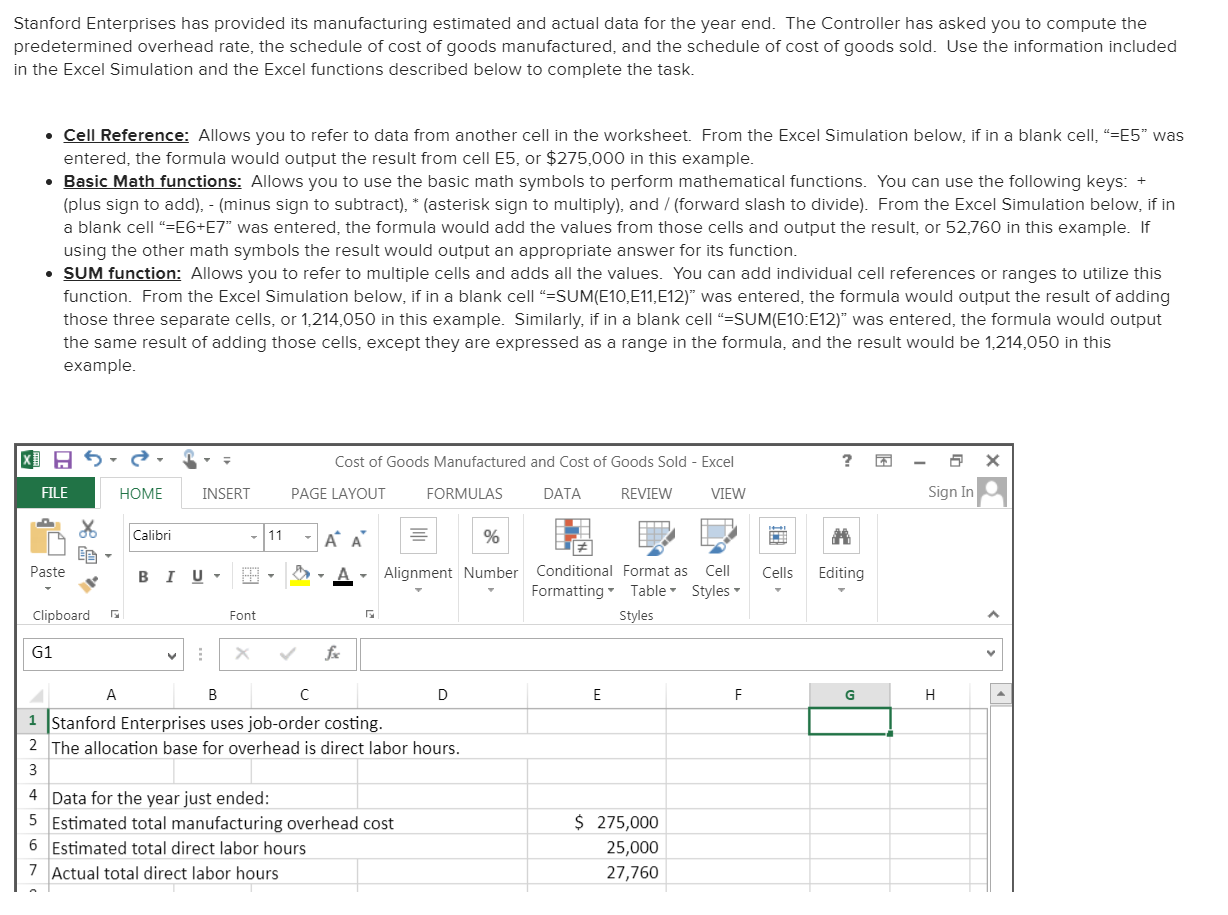Stanford Enterprises has provided its manufacturing estimated and actual data for the year end. The Controller has asked you to compute the predetermined overhead rate, the schedule of cost of goods manufactured, and the schedule of cost of goods sold. Use the information included in the Excel Simulation and the Excel functions described below to complete the task. • Cell Reference: Allows you to refer to data from another cell in the worksheet. From the Excel Simulation below, if in...

• ### Need this tonight please! Deadline: 2/18 11:59p.m Please finish for me. Show calculations necessary, no excel....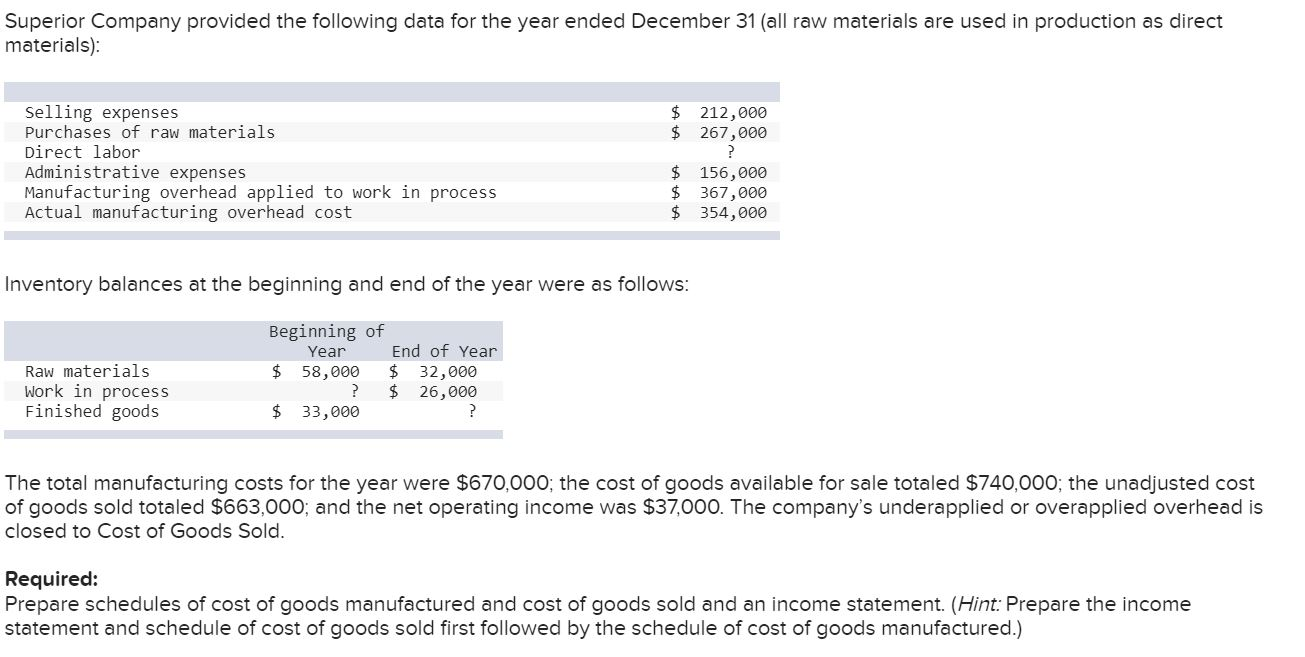Need this tonight please! Deadline: 2/18 11:59p.m Please finish for me. Show calculations necessary, no excel. Thank you. Superior Company provided the following data for the year ended December 31 (all raw materials are used in production as direct materials): \$ \$ 212,000 267,000 Selling expenses Purchases of raw materials Direct labor Administrative expenses Manufacturing overhead applied to work in process Actual manufacturing overhead cost \$ \$ \$ 156,000 367,000 354,000 Inventory balances at the beginning and end of the...

• ### The following cost data relate to the manufacturing activities of Black Company during the just completed...

The following cost data relate to the manufacturing activities of Black Company during the just completed year: Manufacturing overhead costs incurred: Property taxes, factory \$ 2,900 Utilities, factory 4,900 Indirect labor 10,000 Depreciation, factory 23,900 Insurance, factory 5,800 Total actual manufacturing overhead costs \$ 47,500 Other costs incurred: Purchases of raw materials \$ 31,900 Direct labor cost \$ 40,400 Inventories: Raw materials, beginning \$ 8,700 Raw materials, ending \$ 6,700 Work in process, beginning \$ 5,700 Work in process, ending...

Need Online Homework Help?